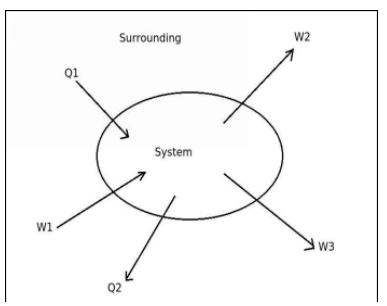# Equation for the First Law of Thermodynamics.## Consider the above diagram of heat transfer and work transfer for a system. What will be the first law equation for the above system?

a. (Q1 – Q2) = ΔE – ( W2 + W3 – W1 )
b. (Q1 + Q2) = ΔE + ( W2 – W3 + W1 )
c. (Q1 – Q2) = ΔE + ( W2 + W3 – W1 )
d. None of the above

Correct Answer : (Q1 – Q2) = ΔE + ( W2 + W3 – W1 )

Explanation :

When a system undergoes cycle, then the algebraic sum of all energy transfer across the boundaries is zero. But when a system undergoes a change of state in which both heat transfer and work transfer are involved, the net energy transfer is stored and collected within the system. If Q is the amount of heat transferred to the system and W is the amount of work transferred from the system during the process, then the net energy (Q – W) is stored in the system. This energy is neither heat nor work but it is called as internal energy(ΔE).
Q – W = ΔE
Therefore,
Q = ΔE + W